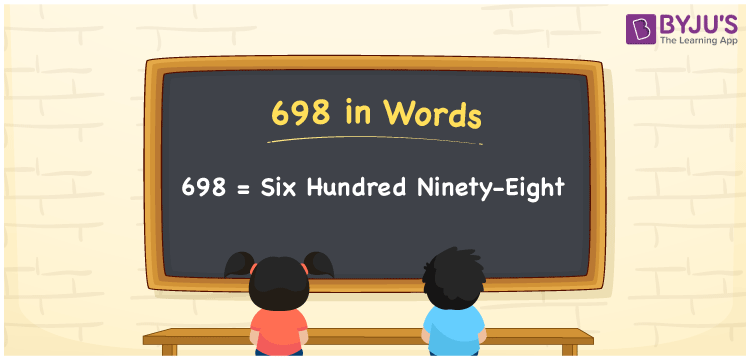# 698 in Words

The number name of 698 is written as “six hundred ninety-eight”. The total cost of 20 notebooks is Rs. 698. It can also be written as “The total cost of twenty notebooks is Rs. Six hundred ninety-eight”. In this article, we will learn the spelling of the number 698 in words using the place value system.

 698 in Words: Six Hundred Ninety-eight. Six Hundred Ninety-eight in Numerical Form: 698.

## 698 in English Words## How to Write 698 in Words?

The place values of 698 are given below:

 Hundreds Tens Ones 6 9 8

The expanded form of 698 is as follows:

= 6 × Hundred + 9 × Ten + 8 × One

= 6 × 100 + 9 × 10 + 8 × 1

= 600 + 90 + 8

= 698

= Six hundred ninety-eight

Hence, 698 in words is six hundred ninety-eight.

698 in words – Six hundred ninety-eight

Is 698 an odd number? – No

Is 698 an even number? – Yes

Is 698 a perfect square number? – No

Is 698 a perfect cube number? – No

Is 698 a prime number? – No

Is 698 a composite number? – Yes

## Frequently Asked Questions on 698 in Words

Q1

### How to write 698 in words?

698 in words is six hundred ninety-eight.

Q2

### Simplify 600 + 98, and express it in words.

Simplifying 600 + 98, we get 698. Hence, 698 in words is six hundred ninety-eight.

Q3

### Is 698 a composite number?

Yes, 698 is a composite number.